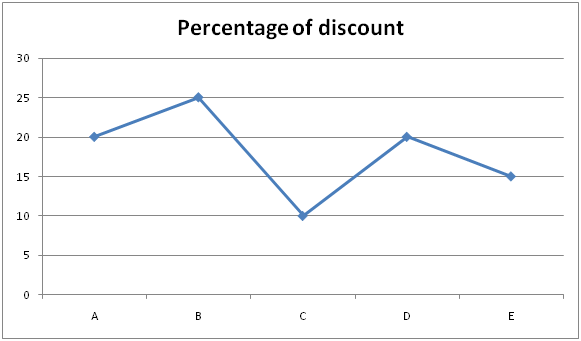# NIACL AO Prelims – Quantitative Aptitude Questions Day- 77

## Quantitative Aptitude for NIACL AO Prelims

Dear Readers, Bank Exam Race for the Year 2019 is already started, To enrich your preparation here we have providing new series of Practice Questions on Quantitative Aptitude – Section. Candidates those who are preparing for NIACL AO Prelims 2019 Exams can practice these questions daily and make your preparation effective.

[WpProQuiz 4877]

Direction (1-5): What will come in place of question mark (?) in the following number series?

1) 12, 36, 90, 180, 270, ?, 135

a) 270

b) 200

c) 190

d) 210

e) 320

2) 50, 53, 60, 73, 92, 121, ?                                                                                                                                                                                                                                  a) 148

b) 158

c) 138

d) 128

e) 118

3) 1, 1, 2, 6, 24, 120, 720, ?

a) 5040

b) 4320

c) 2160

d) 2880

e) 5400

4) 39, 51, ?, 69, 84, 96, 111

a) 60

b) 55

c) 57

d) 58

e) 59

5) 1, 126, 190, 217, 225, ?

a) 250

b) 268

c) 230

d) 226

e) 265

Direction (6 – 10): Study the following information carefully and answer the given questions.

The given line graph shows the percentage of discount offers in five different articles – A, B, C, D and E.6) The shopkeeper purchased an article A and get the discount on its marked price. He sold that article A to customer and made the profit of 25%. In how much percentage above the marked price he sold that article to customer?

a) 20%

b) 28%

c) 36%

d) 15%

e) None of these

7) The marked price of the article C is four-fifth of the marked price of the article D. Ram purchased article C and D and the shopkeeper offer the discount is given value. Sunil also purchased article C and D but the shopkeeper offers the discount of 20% and 10 % respectively. Find the ratio of the total amount paid by Ram and Sunil to the shopkeeper?

a) 66:73

b) 76:77

c) 86:87

d) 66:67

e) None of these

8) The ratio of the marked price of article E and D is 2 : 1. If the profit of article E is Rs. 900 more than that of the profit of article D, then find the marked price of article E?

a) Rs. 4000

b) Rs. 2000

d) Rs. 3000

d) Rs. 5000

e) None of these

9) If the marked price of article A is 50 % more than that of the marked price of article B. The selling price of article A is Rs. 1800 more than that of the selling price article B. If the article B sold at 20 % profit, then find the cost price of the article B?

a) Rs. 2500

b) Rs. 3000

c) Rs. 3600

d) Rs. 4200

e) None of these

10) The ratio of the marked price of article C and D is 50 % and 20 % above the cost price respectively. If the cost price of article C is 150 % more than that of the cost price of the article D, then find the ratio of the selling price of article C to selling price of the article D?

a) 225: 128

b) 223: 141

c) 245: 136

d) 215: 118

e) None of these

Direction (1-5) :

12*3=36

36*2.5=90

90*2=180

180*1.5=270

270*1=270

270*0.5=135

50+3=53

53+7=60

60+13=73

73+19=92

92+29=121

121+37=158

1*1=1

1*2=2

2*3=6

6*4=24

24*5=120

120*6=720

720*7=5040

39+3+9=51

51+5+1=57

57+5+7=69

69+6+9=84

84+8+4=96

96+9+6=111

1+53=126

126+43=190

190+33=217

217+23=225

225+13=226

Direction (6-10) :

Let the MP=Rs.100x

SP =100x*(80/100) =80x

80x=CP*(125/100)

CP = 64x

Required percentage= [(100x – 64x)/100x]*100=36%

Let the MP of D=100x

MP of C = (4/5)*100x=80x

Ram=80x*90/100 + 100x*80/100 = 72x+80x=152x

Sunil=80x*80/100 + 100x*90/100 = 64x+90x=154x

Required ratio=152x: 154=76: 77

MP of the article E = 2x

MP of the article D = x

2x*85/100 – x*80/100 = 900

90x = 90000

X = Rs. 1000

MP of article E = 2*(1000) = Rs. 2000

CP of article B = x

MP of article B = y

SP of article B = y*75/100 = 3y/4

MP of article A = (150/100)*y = 3y/2

SP of article A = (3y/2)*(80/100) = 6y/5

6y/5 – 3y/4 = 1800

24y – 15y = 1800*20

Y = Rs. 4000

According to the question,

4000*(75/100) = x*(120/100)

X = Rs. 2500

CP of D = x

CP of C = (250/100)*x = 5x/4

MP of C = (5x/4)*(150/100) = 15x/8

MP of D = x*(120/100) = 6x/5

Required ratio= [(15x/8)*(90/100)] : [(6x/5)*(80/100)] = 225 : 128